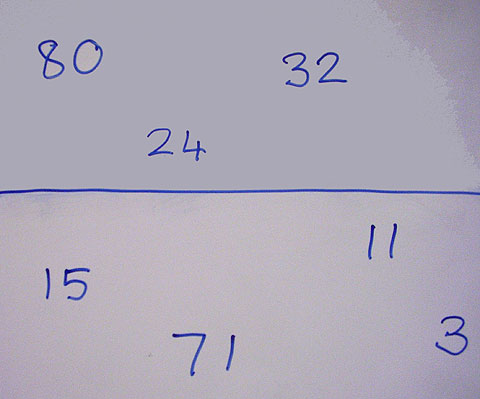Home > Reasoning > Activities > Above and below the line

# Above and below the line

Draw a horizontal line across the middle of the board.

Without speaking, write 24, 32 and 80 (or other multiples of 4) above the line and 15, 11 and 71 below the line.

Explain that the top numbers have a 'rule' and ask students if they know another number to write either above or below the line.Starting point for activity.

Invite individuals to write their numbers on the board. When most of the students seem to know 'the rule', ask some individuals what they think the rule is. Go back to some of the answers that were sensible but wrong. For example, some students may have thought that the rule was 'evens above and odds below'.

Ask students to add other numbers, challenging and extending knowledge. For example: "Nobody has added a negative number yet," or "We need some numbers that are greater than 100."

Focus the discussion on reasoning. Some possible questions are listed below.

• What is common about the numbers above the line?
• How do you know 12 belongs above the line?
• Can you see anything that all the multiples of four have in common? Are there any numbers that end in 2 that are not multiples of 4, to put below the line?
• Can a number ending in 3 go above the line? Why not?
• If I had this number (3407) where would it go, and why?

This activity may be used at all year levels with various categories of number (including fractions and types of integers), measurement units, and shapes. It could be extended to include a third and fourth classification area. You can download some suggestions for primary students in 'Above and Below the Line' Ideas, F–6.

## Curriculum links

Year 1: Investigate and describe number patterns formed by skip counting and patterns with objects

Year 4: Investigate number sequences involving multiples of 3, 4, 6, 7, 8, and 9

Year 1: Investigate and describe number patterns formed by skip counting and patterns with objects

Year 4: Investigate number sequences involving multiples of 3, 4, 6, 7, 8, and 9

Source